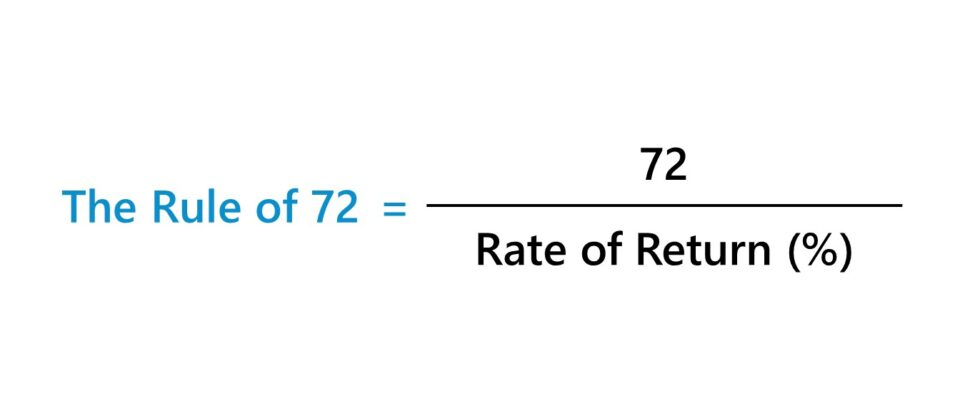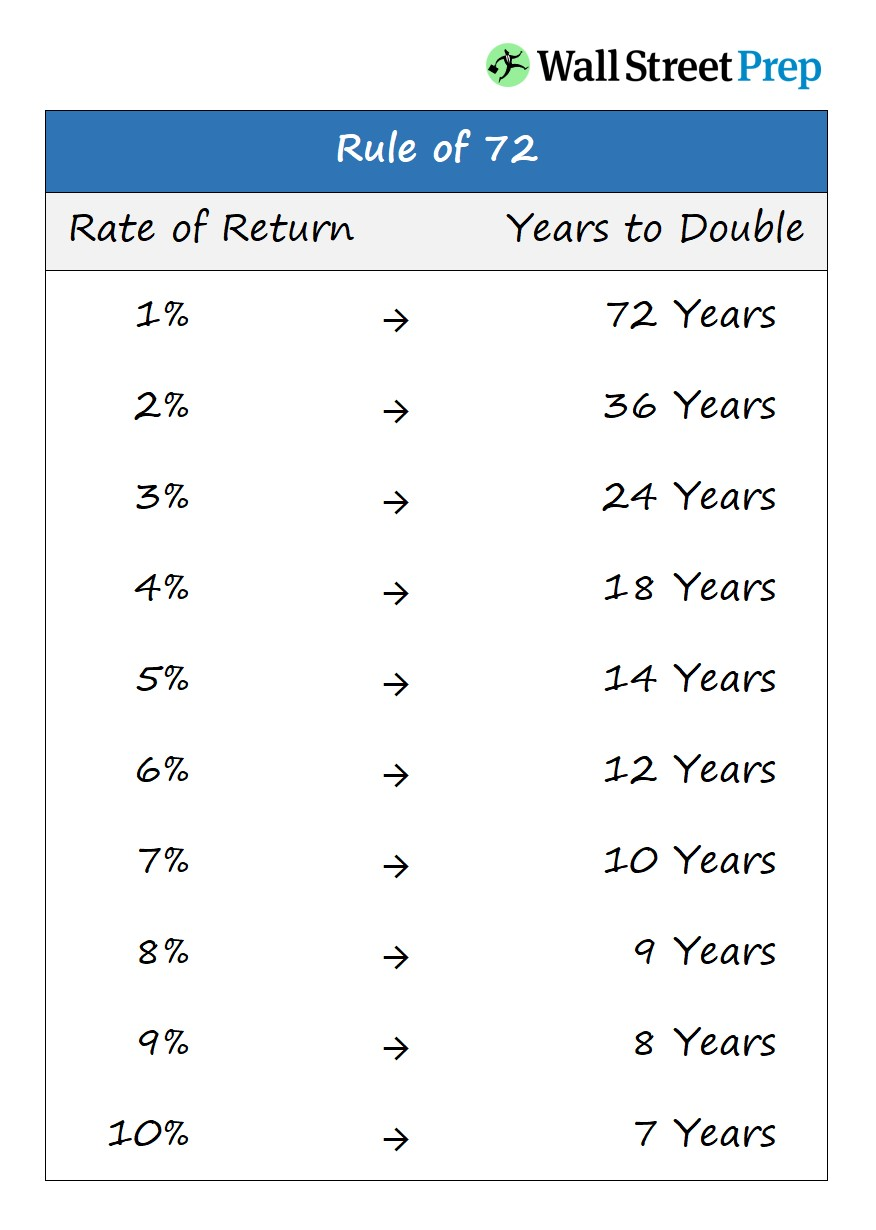Welcome to Wall Street Prep! Use code at checkout for 15% off.# The Rule of 72

Guide to Understanding the Rule of 72## How to Calculate Rule of 72?

The Rule of 72 is a convenient approach to approximate how long it will take for invested capital to double in value.

In order to figure out the number of years it would take to double an investment, 72 is divided by the investment’s annual return.

The calculation is more so a rough estimate – i.e. “back of the envelope” math – that provides a relatively accurate figure.

For a more precise figure, using Excel (or a financial calculator) is recommended.

The Rule of 72 is well-known in finance and perceived by most as a general rule of thumb to estimate the number of years that it would take an investment to double in value.

Yet, in spite of the simplicity of the calculation and the convenience, the methodology is rather accurate, within a reasonable range.

## Rule of 72 Formula

The formula for the Rule of 72 divides the number 72 by the annualized rate of return (i.e. the interest rate).

Number of Years to Double = 72 ÷ Interest Rate (%)

Thus, the implied number of years for the investment’s value to double (2x) can be approximated by dividing the number 72 by the effective interest rate. However, the effective interest rate used in the equation is not in percentage form.

For example, if an investor decided to contribute \$200,000 to an active investor’s fund.

According to the firm’s marketing documents, the normalized return should range around 9% approximately, i.e. the 9% is the set return targeted by the fund’s portfolio of investments over the long term (and various economic cycles).

If we assume the 9% annual return is in fact achieved, the estimated number of years for the original investment to double in value is roughly 8 years.

• Number of Years to Double (n) = 72 ÷ 9 = 8 Years

## Rule of 72 Chart: Implied Number of Years to Double

The chart below provides the approximate number of years for an investment to double.

The left column lists the rate of return – from 1% to 10% – while the right column states the number of years it would take for the investment to double in value based on the corresponding return.## The Rule of 72: Compound Interest vs. Simple Interest

The Rule of 72 applies to cases of compound interest, but not to simple interest.

• Simple Interest – The accumulated interest to date is NOT added back to the original principal amount.
• Compound Interest – The interest is calculated based on the original principal, as well as the accumulated interest incurred from prior periods (i.e. “interest on interest”).

## Rule of 72 Calculator

We’ll now move to a modeling exercise, which you can access by filling out the form below.Submitting...

## 1. Rule of 72 Calculation Example

Let’s say, for example, an investment is earning 6% each year.

If we divide 72 by 6, we can calculate the number of years it would take for the investment to double.

• Implied Number of Years to Double (2x) = 72 ÷ 6 = 12 Years

In our illustrative scenario, the investment needs around 12 years before doubling in value.

## 2. Rule of 115 Calculation Example

There is also a related but lesser-known rule, called the “Rule of 115”.

Number of Years to Triple = 115 ÷ Interest Rate (%)

By dividing 115 by the rate of return, the estimated time for an investment to triple (3x) can be calculated.

Continuing off the previous example with the 6% return assumption:

• Implied Number of Years to Triple (3x) = 115 ÷ 6 = 19 YearsMaster LBO Modeling Our Advanced LBO Modeling course will teach you how to build a comprehensive LBO model and give you the confidence to ace the finance interview.Inline Feedbackscalcuing
March 25, 2022 7:24 am

Rule of 72 formula offer you to have simple calculation where you can solve your equation of doubling the investment time period.March 25, 2022 11:36 am

Yes, the Rule of 72 allows you to estimate the amount of time it will take to double by dividing by the rate of return.Learn Financial Modeling Online

Everything you need to master financial and valuation modeling: 3-Statement Modeling, DCF, Comps, M&A and LBO.

X

The Wall Street Prep Quicklesson Series

7 Free Financial Modeling Lessons

Get instant access to video lessons taught by experienced investment bankers. Learn financial statement modeling, DCF, M&A, LBO, Comps and Excel shortcuts.